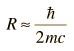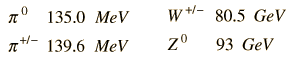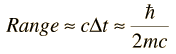# Exchange Forces

All four of the fundamental forces involve the exchange of one or more particles. Even the underlying color force which is presumed to hold the quarks together to make up the range of observed particles involves an exchange of particles labeled gluons.
Such exchange forces may be either attractive or repulsive, but are limited in range by the nature of the exchange force. The maximum range of an exchange force is dictated by the uncertainty principle since the particles involved are created and exist only in the exchange process - they are called "virtual" particles. Such exchange forces are often pictured with Feynman diagrams.

### graviton

Index

Fundamental force concepts

 HyperPhysics***** Quantum Physics R Nave
Go Back

# Range of Forces

If a force involves the exchange of a particle, that particle has to "get back home before it is missed" in the sense that it must fit within the constraints of the uncertainty principle. A particle of mass m and rest energy E=mc2 can be exchanged if it does not go outside the bounds of the uncertainty principle in the formA particle which can exist only within the constraints of the uncertainty principle is called a "virtual particle", and the time in the expression above represents the maximum lifetime of the virtual exchange particle. Since this exchange particle cannot exceed the speed limit of the universe, it cannot travel further than c times that lifetime. The maximum range of the force would then be on the order ofNote that this expression implies that a zero mass for the exchange particle implies a force of infinite range. The rest masses of the exchange particles for the electromagnetic force and gravity, the photon and the graviton, are taken to be zero and those forces are presumed to be infinite in range. The recent detection of gravity waves is consistent with transmission at the speed of light and therefore with a graviton mass of zero.

### Calculation

Index

Fundamental force concepts

 HyperPhysics***** Quantum Physics R Nave
Go Back

# Range of Exchange Force

Using the approximate range expression arising from the uncertainty principle and the speed of light, an exchange particle of mass

m = x10^ kg = MeV/c2 = GeV/c2
 m = melectron = mproton

 could give a range of:

Range = x10^ m = fermi* = proton radii**.

The range expression can be explored numerically by entering masses or rest mass energies, like those of the pions below. A range can be entered to calculate the exchange particle mass associated with such a range.* 1 fermi = 10-15 meters.
**calculated radius using nominal nuclear density 2.3 x 1017 kg/m3.
Index

Fundamental force concepts

 HyperPhysics***** Quantum Physics R Nave
Go Back

# Pion Range of Strong Force

An estimate of the range of the strong force can be made by assuming that it is an exchange force involving neutral pions. This would be considering the pion involved in the exchange to be a "virtual particle", limited in lifetime by the uncertainty principle. When the range expression### Calculation

is used with a pion mass of

π0 mass = 264 me = 135.0 MeV/c2
the range obtained is
Range = 0.73 x 10-15 m = 0.61 x classical proton radius.

This range is in the neighborhood of one fermi. When Hideki Yukawa was working on a theory of the strong force, he judged that the range of the nuclear force was about a fermi, and calculated that the exchange particle should be in the neighborhood of 100 MeV in mass equivalent. This triggered the search which led to the discovery of the pion. When the nuclear particles are very close together, other heavier particles must also be included in this type of model of the strong force. The current view is that the strong force is fundamentally an interaction between quarks, called the "color force" and that the "strong force" between nucleons which are colorless is really a residual color force.

Inside a proton or neutron (or any hadron), the force between quarks does not decrease with distance, leading to the confinement of quarks. But outside a proton or neutron, the strong force between them drops off precipitously within about a fermi of distance. The pion range is a reasonable predictor of this precipitous drop and gives further insight into the paradoxical nature of the strong nuclear force. For a proton to attract a neighboring proton, it must exchange something with it, but an isolated quark cannot be exchanged because of quark confinement. However, it can exchange a quark-antiquark pair (a meson) and the pion is the lightest of the mesons. Lighter exchange particle implies longer range, so the pion range gives you an upper bound for an exchange force involving quark-antiquark pairs.

Index

Fundamental force concepts

 HyperPhysics***** Quantum Physics R Nave
Go Back